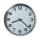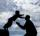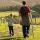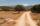Pedestrian and cyclist

Pedestrian out with a speed of 4 km/hour from city center and after 1hour and 10 minutes came after him cyclist at speed of 18 km/h. At how many minutes he catches up with pedestrian?

Result

t =  20 min

Solution:

4(t+(1*60+10))/60 = 18t/60

14t = 280

t = 20

Calculated by our simple equation calculator.

Leave us a comment of this math problem and its solution (i.e. if it is still somewhat unclear...):Be the first to comment!To solve this verbal math problem are needed these knowledge from mathematics:

Do you have a linear equation or system of equations and looking for its solution? Or do you have quadratic equation?

Next similar math problems:

1. Find xSolve: if 2(x-1)=14, then x= (solve an equation with one unknown)
2. Two cars 2Two cars started from two positions 87 km distant at the same time in opposite directions at speeds 81 km/h and 75 km/h. What was the distance between them after 2 hours 50 minutes of driving.
3. Dropped sheetsThree consecutive sheets dropped from the book. The sum of the numbers on the pages of the dropped sheets is 273. What number has the last page of the dropped sheets?
4. HoursThe lesson lasts 45 minutes. For the week, students have 18 lesson hours. How many are the actual hours?
5. ClockHow many hours are 15 days?
6. SuzanSusan's age will be after 12 years four times as much as twelve years ago. How old is Susan now?
7. TimeageSeven times of my age is 8 less than the largest two-digit number. How old I am?
8. Family 8Father is 38 years old, daughter 12, son 14. How many years will father have as many years as his children together?
9. NormThree workers planted 3555 seedlings of tomatoes in one dey. First worked at the standard norm, the second planted 120 seedlings more and the third 135 seedlings more than the first worker. How many seedlings were standard norm?
10. Equation 29Solve next equation: 2 ( 2x + 3 ) = 8 ( 1 - x) -5 ( x -2 )
11. Father and sonFather is 44 years old, his son 16 years. Determine how many years ago was the father five times older than the son.
12. Six te 2If 3t-7=5t, then 6t=
13. Negative in equation2x + 3 + 7x = – 24, what is the value of x?Daddy is a 46 year old and son is at age of 16. When (which year) was/will daddy 5 times more years than his son?Five mates traveled and the journey they lasted 195 minutes. How long would travel only one of them?Solve for x: 3(x + 2) = x - 18Honza had three cages (black, silver, gold) and three animals (guinea pig, rat and puppy). There was one animal in each cage. The golden cage stood to the left of the black cage. The silver cage stood on the right of the guinea pig cage. The rat was in the